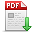# 胡春鹤,王依帆,朱书豪,等.基于鸽群优化算法的图像分割方法研究[J].郑州大学学报(工学版),2019,40(04):8. 　Research on Image Segmentation Method Based on Pigeon Group Optimization Algorithm[J].Journal of Zhengzhou University (Engineering Science),2019,40(04):8. 点击复制 基于鸽群优化算法的图像分割方法研究() 分享到： var jiathis_config = { data_track_clickback: true };

40

2019年04期

8

2019-07-10

## 文章信息/Info

Title:
Research on Image Segmentation Method Based on Pigeon Group Optimization Algorithm

A

Abstract:
Image segmentation is a kind of constrained nonlinear optimization problem that needs to seek the optimal solution in nonlinear parameter space. In order to improve the precision of the optimization problem, an image segmentation method based on pigeon group optimization algorithm is proposed. First, the segmentation threshold is used as the optimization variable, and the image segmentation is modeled as a nonlinear optimization problem with the optimal threshold equation as the objective function, and the inter-class variance and the w 0 and w1 ranges as the constraints. Then, Using random segmentation threshold as the initial value of iteration, the optimal parameters are solved by the pigeon group optimization algorithm(PIO). In order to verify the validity of this method, two kinds of images with different features are divided into experiments, and the algorithm is evaluated by overlapping degree and time efficiency. The results show that the algorithm has the highest degree of overlap and the shortest operation time.